Next: General resolution Up: Affine-to-Euclidean calibration Previous: Affine-to-Euclidean calibration

### Resolution of eq:kkt

As we have seen previously, given an infinite homography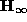between the images of the same camera, it is possible to calculate its real Jordan decomposition and hence a matrix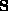such that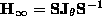. Then, it was shown thatshould satisfy eq:kkt. The calibration process consists of solving this equation which can be written as a system of 6 equations with 7 unknowns (a, k,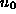,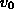, r,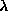and) :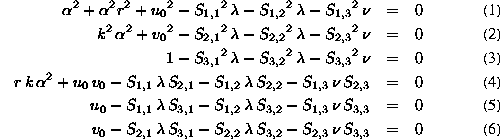It is clear that this system cannot be solved just as it is (there is one unknown too many). We must either consider a constraint on one unknown, or add equations from several motions (with non parallel rotation axes).

If we want to calibrate with a single motion or with planar motions (where all rotation axes are parallel), a constraint on the internal parameters must be imposed. Commonly used constraints are either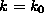or r=0.

Bob Fisher
Mon Dec 7 13:48:06 GMT 1998